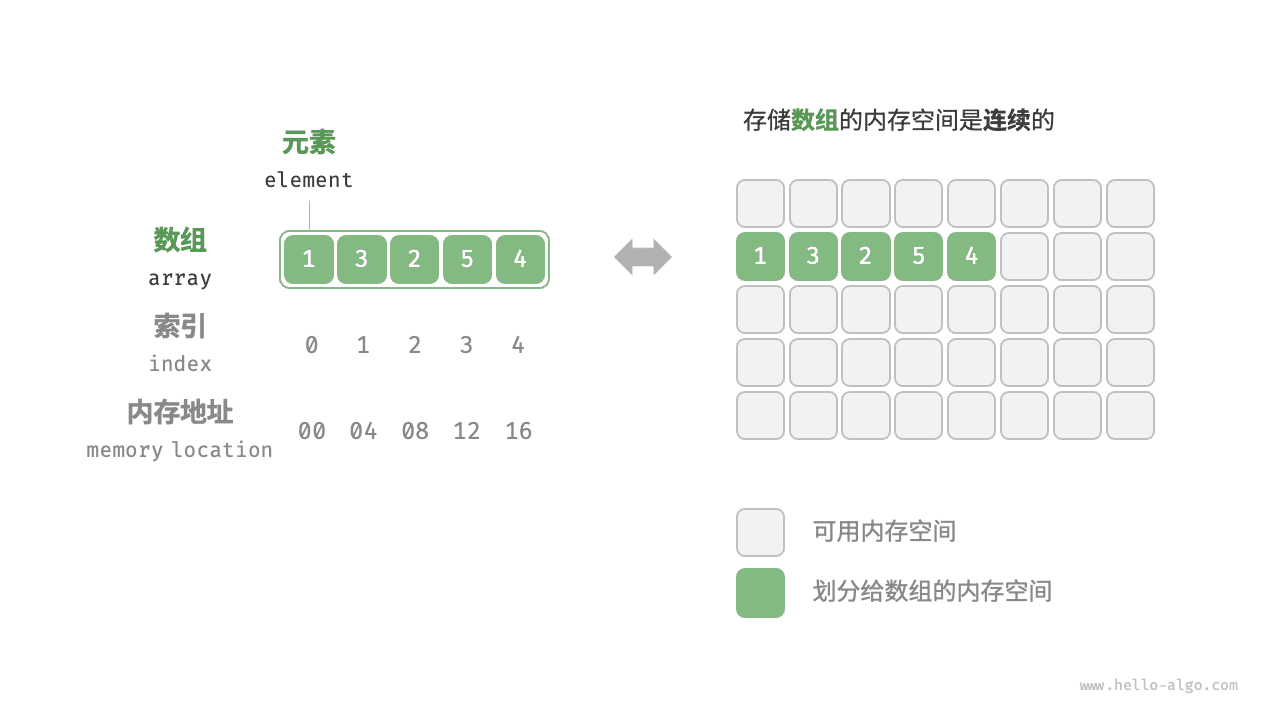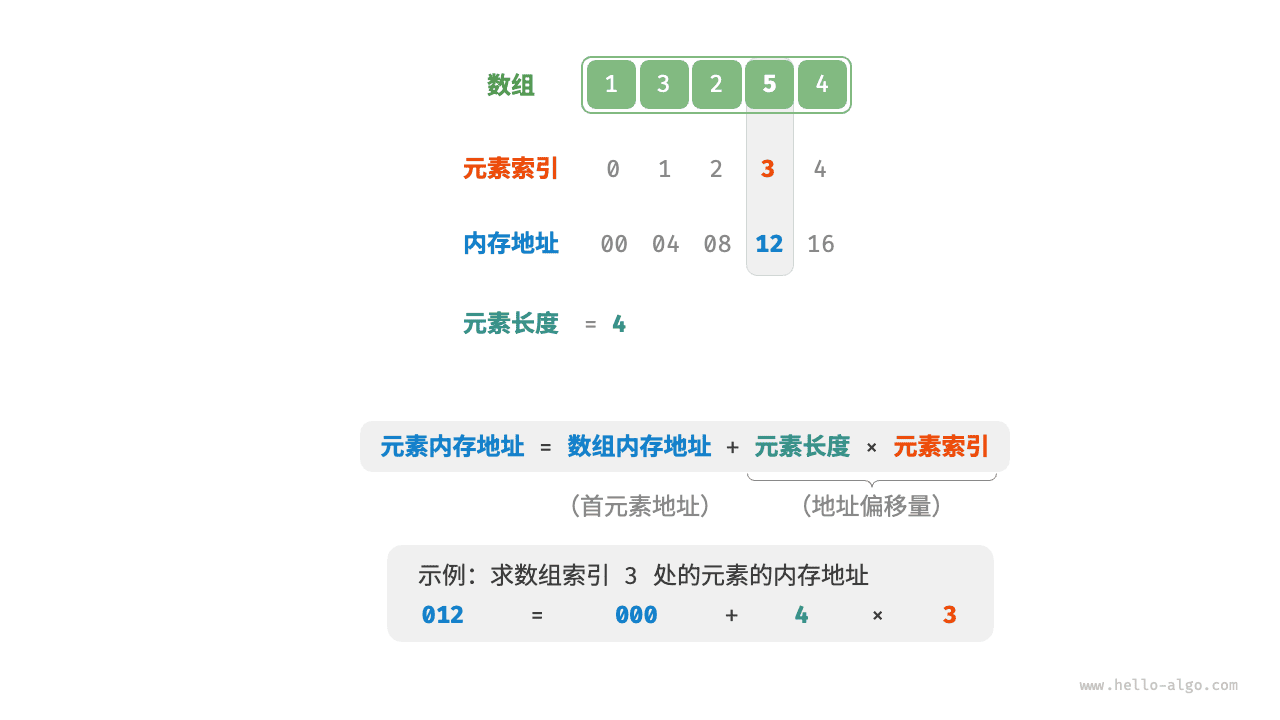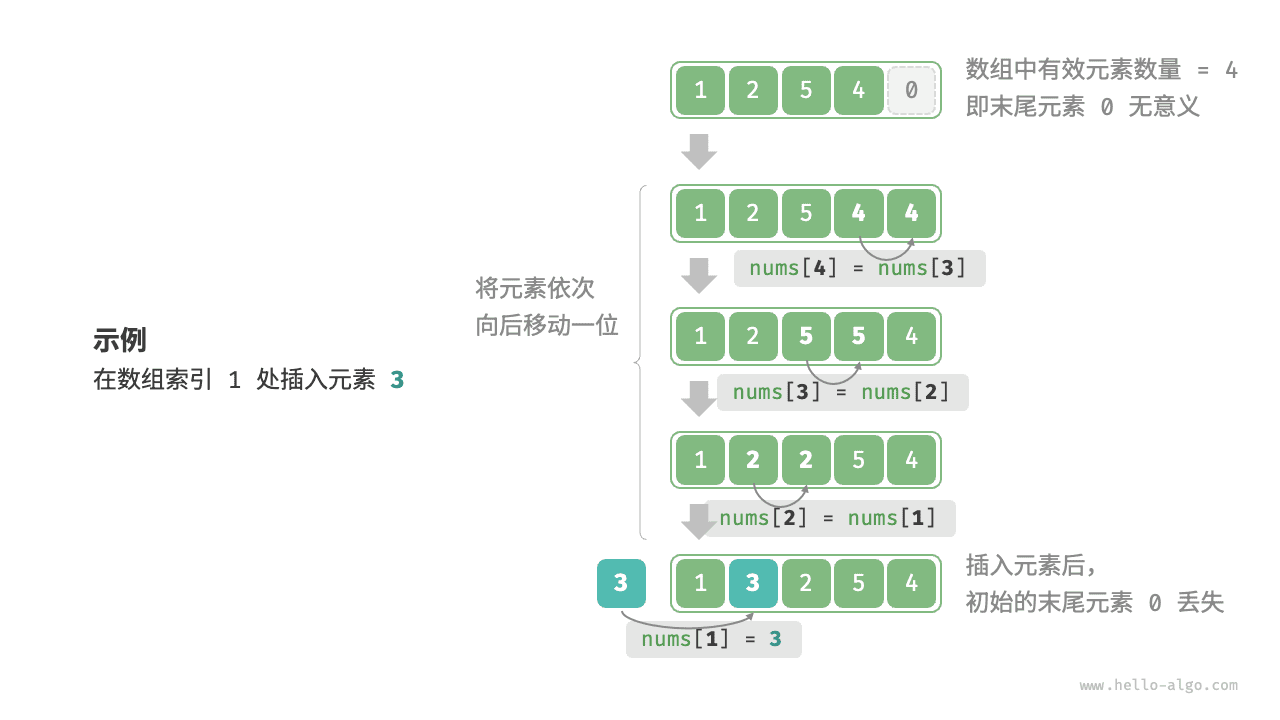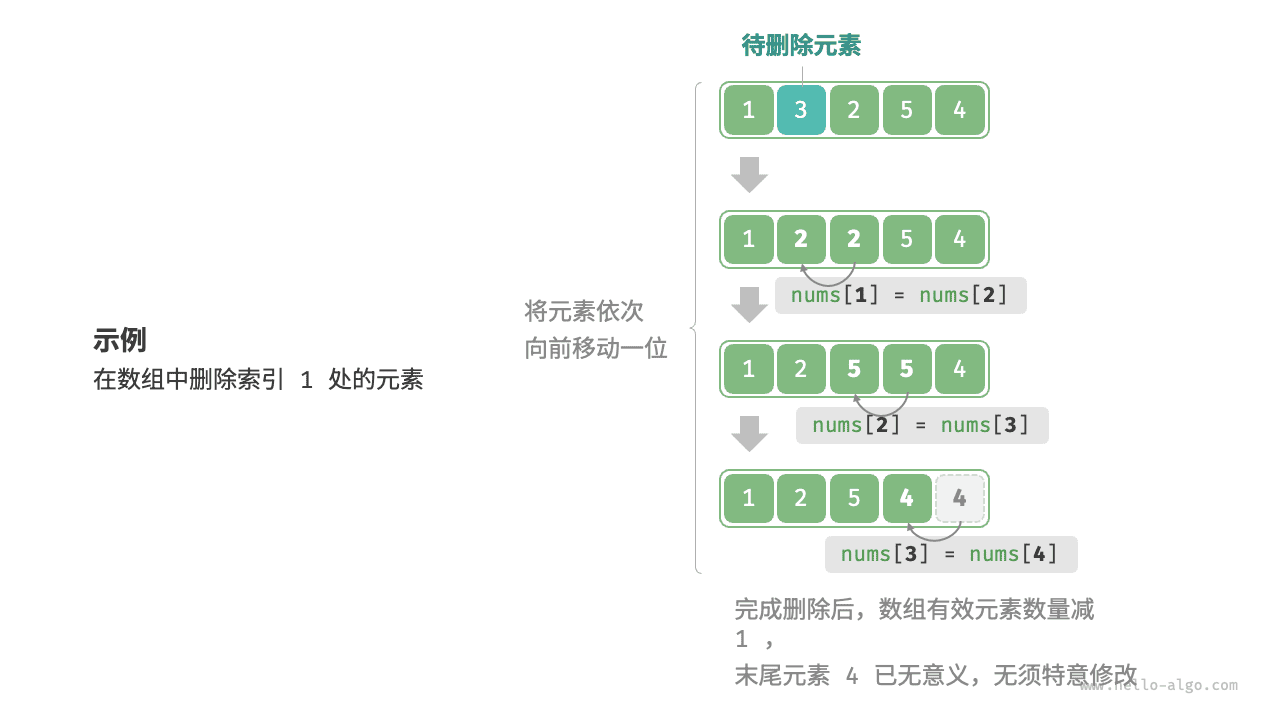# 4.1   数组¶

「数组 array」是一种线性数据结构，其将相同类型的元素存储在连续的内存空间中。我们将元素在数组中的位置称为该元素的「索引 index」。图 4-1 展示了数组的主要术语和概念。## 4.1.1   数组常用操作¶

### 1.   初始化数组¶

array.py
# 初始化数组
arr: list[int] =  * 5  # [ 0, 0, 0, 0, 0 ]
nums: list[int] = [1, 3, 2, 5, 4]

array.cpp
/* 初始化数组 */
// 存储在栈上
int arr;
int nums = { 1, 3, 2, 5, 4 };
// 存储在堆上（需要手动释放空间）
int* arr1 = new int;
int* nums1 = new int { 1, 3, 2, 5, 4 };

array.java
/* 初始化数组 */
int[] arr = new int; // { 0, 0, 0, 0, 0 }
int[] nums = { 1, 3, 2, 5, 4 };

array.cs
/* 初始化数组 */
int[] arr = new int; // { 0, 0, 0, 0, 0 }
int[] nums = [1, 3, 2, 5, 4];

array.go
/* 初始化数组 */
var arr int
// 在 Go 中，指定长度时（int）为数组，不指定长度时（[]int）为切片
// 由于 Go 的数组被设计为在编译期确定长度，因此只能使用常量来指定长度
// 为了方便实现扩容 extend() 方法，以下将切片（Slice）看作数组（Array）
nums := []int{1, 3, 2, 5, 4}

array.swift
/* 初始化数组 */
let arr = Array(repeating: 0, count: 5) // [0, 0, 0, 0, 0]
let nums = [1, 3, 2, 5, 4]

array.js
/* 初始化数组 */
var arr = new Array(5).fill(0);
var nums = [1, 3, 2, 5, 4];

array.ts
/* 初始化数组 */
let arr: number[] = new Array(5).fill(0);
let nums: number[] = [1, 3, 2, 5, 4];

array.dart
/* 初始化数组 */
List<int> arr = List.filled(5, 0); // [0, 0, 0, 0, 0]
List<int> nums = [1, 3, 2, 5, 4];

array.rs
/* 初始化数组 */
let arr: Vec<i32> = vec![0; 5]; // [0, 0, 0, 0, 0]
let nums: Vec<i32> = vec![1, 3, 2, 5, 4];

array.c
/* 初始化数组 */
int arr = { 0 }; // { 0, 0, 0, 0, 0 }
int nums = { 1, 3, 2, 5, 4 };

array.zig
// 初始化数组
var arr = [_]i32{0} ** 5; // { 0, 0, 0, 0, 0 }
var nums = [_]i32{ 1, 3, 2, 5, 4 };


### 2.   访问元素¶array.py
def random_access(nums: list[int]) -> int:
"""随机访问元素"""
# 在区间 [0, len(nums)-1] 中随机抽取一个数字
random_index = random.randint(0, len(nums) - 1)
# 获取并返回随机元素
random_num = nums[random_index]
return random_num

array.cpp
/* 随机访问元素 */
int randomAccess(int *nums, int size) {
// 在区间 [0, size) 中随机抽取一个数字
int randomIndex = rand() % size;
// 获取并返回随机元素
int randomNum = nums[randomIndex];
return randomNum;
}

array.java
/* 随机访问元素 */
int randomAccess(int[] nums) {
// 在区间 [0, nums.length) 中随机抽取一个数字
// 获取并返回随机元素
int randomNum = nums[randomIndex];
return randomNum;
}

array.cs
/* 随机访问元素 */
int RandomAccess(int[] nums) {
Random random = new();
// 在区间 [0, nums.Length) 中随机抽取一个数字
int randomIndex = random.Next(nums.Length);
// 获取并返回随机元素
int randomNum = nums[randomIndex];
return randomNum;
}

array.go
/* 随机访问元素 */
func randomAccess(nums []int) (randomNum int) {
// 在区间 [0, nums.length) 中随机抽取一个数字
randomIndex := rand.Intn(len(nums))
// 获取并返回随机元素
randomNum = nums[randomIndex]
return
}

array.swift
/* 随机访问元素 */
func randomAccess(nums: [Int]) -> Int {
// 在区间 [0, nums.count) 中随机抽取一个数字
let randomIndex = nums.indices.randomElement()!
// 获取并返回随机元素
let randomNum = nums[randomIndex]
return randomNum
}

array.js
/* 随机访问元素 */
function randomAccess(nums) {
// 在区间 [0, nums.length) 中随机抽取一个数字
const random_index = Math.floor(Math.random() * nums.length);
// 获取并返回随机元素
const random_num = nums[random_index];
return random_num;
}

array.ts
/* 随机访问元素 */
function randomAccess(nums: number[]): number {
// 在区间 [0, nums.length) 中随机抽取一个数字
const random_index = Math.floor(Math.random() * nums.length);
// 获取并返回随机元素
const random_num = nums[random_index];
return random_num;
}

array.dart
/* 随机访问元素 */
int randomAccess(List<int> nums) {
// 在区间 [0, nums.length) 中随机抽取一个数字
int randomIndex = Random().nextInt(nums.length);
// 获取并返回随机元素
int randomNum = nums[randomIndex];
return randomNum;
}

array.rs
/* 随机访问元素 */
fn random_access(nums: &[i32]) -> i32 {
// 在区间 [0, nums.len()) 中随机抽取一个数字
// 获取并返回随机元素
let random_num = nums[random_index];
random_num
}

array.c
/* 随机访问元素 */
int randomAccess(int *nums, int size) {
// 在区间 [0, size) 中随机抽取一个数字
int randomIndex = rand() % size;
// 获取并返回随机元素
int randomNum = nums[randomIndex];
return randomNum;
}

array.zig
// 随机访问元素
fn randomAccess(nums: []i32) i32 {
// 在区间 [0, nums.len) 中随机抽取一个整数
var randomIndex = std.crypto.random.intRangeLessThan(usize, 0, nums.len);
// 获取并返回随机元素
var randomNum = nums[randomIndex];
return randomNum;
}


### 3.   插入元素¶array.py
def insert(nums: list[int], num: int, index: int):
"""在数组的索引 index 处插入元素 num"""
# 把索引 index 以及之后的所有元素向后移动一位
for i in range(len(nums) - 1, index, -1):
nums[i] = nums[i - 1]
# 将 num 赋给 index 处的元素
nums[index] = num

array.cpp
/* 在数组的索引 index 处插入元素 num */
void insert(int *nums, int size, int num, int index) {
// 把索引 index 以及之后的所有元素向后移动一位
for (int i = size - 1; i > index; i--) {
nums[i] = nums[i - 1];
}
// 将 num 赋给 index 处的元素
nums[index] = num;
}

array.java
/* 在数组的索引 index 处插入元素 num */
void insert(int[] nums, int num, int index) {
// 把索引 index 以及之后的所有元素向后移动一位
for (int i = nums.length - 1; i > index; i--) {
nums[i] = nums[i - 1];
}
// 将 num 赋给 index 处的元素
nums[index] = num;
}

array.cs
/* 在数组的索引 index 处插入元素 num */
void Insert(int[] nums, int num, int index) {
// 把索引 index 以及之后的所有元素向后移动一位
for (int i = nums.Length - 1; i > index; i--) {
nums[i] = nums[i - 1];
}
// 将 num 赋给 index 处的元素
nums[index] = num;
}

array.go
/* 在数组的索引 index 处插入元素 num */
func insert(nums []int, num int, index int) {
// 把索引 index 以及之后的所有元素向后移动一位
for i := len(nums) - 1; i > index; i-- {
nums[i] = nums[i-1]
}
// 将 num 赋给 index 处的元素
nums[index] = num
}

array.swift
/* 在数组的索引 index 处插入元素 num */
func insert(nums: inout [Int], num: Int, index: Int) {
// 把索引 index 以及之后的所有元素向后移动一位
for i in nums.indices.dropFirst(index).reversed() {
nums[i] = nums[i - 1]
}
// 将 num 赋给 index 处的元素
nums[index] = num
}

array.js
/* 在数组的索引 index 处插入元素 num */
function insert(nums, num, index) {
// 把索引 index 以及之后的所有元素向后移动一位
for (let i = nums.length - 1; i > index; i--) {
nums[i] = nums[i - 1];
}
// 将 num 赋给 index 处的元素
nums[index] = num;
}

array.ts
/* 在数组的索引 index 处插入元素 num */
function insert(nums: number[], num: number, index: number): void {
// 把索引 index 以及之后的所有元素向后移动一位
for (let i = nums.length - 1; i > index; i--) {
nums[i] = nums[i - 1];
}
// 将 num 赋给 index 处的元素
nums[index] = num;
}

array.dart
/* 在数组的索引 index 处插入元素 _num */
void insert(List<int> nums, int _num, int index) {
// 把索引 index 以及之后的所有元素向后移动一位
for (var i = nums.length - 1; i > index; i--) {
nums[i] = nums[i - 1];
}
// 将 _num 赋给 index 处元素
nums[index] = _num;
}

array.rs
/* 在数组的索引 index 处插入元素 num */
fn insert(nums: &mut Vec<i32>, num: i32, index: usize) {
// 把索引 index 以及之后的所有元素向后移动一位
for i in (index + 1..nums.len()).rev() {
nums[i] = nums[i - 1];
}
// 将 num 赋给 index 处的元素
nums[index] = num;
}

array.c
/* 在数组的索引 index 处插入元素 num */
void insert(int *nums, int size, int num, int index) {
// 把索引 index 以及之后的所有元素向后移动一位
for (int i = size - 1; i > index; i--) {
nums[i] = nums[i - 1];
}
// 将 num 赋给 index 处的元素
nums[index] = num;
}

array.zig
// 在数组的索引 index 处插入元素 num
fn insert(nums: []i32, num: i32, index: usize) void {
// 把索引 index 以及之后的所有元素向后移动一位
var i = nums.len - 1;
while (i > index) : (i -= 1) {
nums[i] = nums[i - 1];
}
// 将 num 赋给 index 处的元素
nums[index] = num;
}


### 4.   删除元素¶array.py
def remove(nums: list[int], index: int):
"""删除索引 index 处的元素"""
# 把索引 index 之后的所有元素向前移动一位
for i in range(index, len(nums) - 1):
nums[i] = nums[i + 1]

array.cpp
/* 删除索引 index 处的元素 */
void remove(int *nums, int size, int index) {
// 把索引 index 之后的所有元素向前移动一位
for (int i = index; i < size - 1; i++) {
nums[i] = nums[i + 1];
}
}

array.java
/* 删除索引 index 处的元素 */
void remove(int[] nums, int index) {
// 把索引 index 之后的所有元素向前移动一位
for (int i = index; i < nums.length - 1; i++) {
nums[i] = nums[i + 1];
}
}

array.cs
/* 删除索引 index 处的元素 */
void Remove(int[] nums, int index) {
// 把索引 index 之后的所有元素向前移动一位
for (int i = index; i < nums.Length - 1; i++) {
nums[i] = nums[i + 1];
}
}

array.go
/* 删除索引 index 处的元素 */
func remove(nums []int, index int) {
// 把索引 index 之后的所有元素向前移动一位
for i := index; i < len(nums)-1; i++ {
nums[i] = nums[i+1]
}
}

array.swift
/* 删除索引 index 处的元素 */
func remove(nums: inout [Int], index: Int) {
// 把索引 index 之后的所有元素向前移动一位
for i in nums.indices.dropFirst(index).dropLast() {
nums[i] = nums[i + 1]
}
}

array.js
/* 删除索引 index 处的元素 */
function remove(nums, index) {
// 把索引 index 之后的所有元素向前移动一位
for (let i = index; i < nums.length - 1; i++) {
nums[i] = nums[i + 1];
}
}

array.ts
/* 删除索引 index 处的元素 */
function remove(nums: number[], index: number): void {
// 把索引 index 之后的所有元素向前移动一位
for (let i = index; i < nums.length - 1; i++) {
nums[i] = nums[i + 1];
}
}

array.dart
/* 删除索引 index 处的元素 */
void remove(List<int> nums, int index) {
// 把索引 index 之后的所有元素向前移动一位
for (var i = index; i < nums.length - 1; i++) {
nums[i] = nums[i + 1];
}
}

array.rs
/* 删除索引 index 处的元素 */
fn remove(nums: &mut Vec<i32>, index: usize) {
// 把索引 index 之后的所有元素向前移动一位
for i in index..nums.len() - 1 {
nums[i] = nums[i + 1];
}
}

array.c
/* 删除索引 index 处的元素 */
// 注意：stdio.h 占用了 remove 关键词
void removeItem(int *nums, int size, int index) {
// 把索引 index 之后的所有元素向前移动一位
for (int i = index; i < size - 1; i++) {
nums[i] = nums[i + 1];
}
}

array.zig
// 删除索引 index 处的元素
fn remove(nums: []i32, index: usize) void {
// 把索引 index 之后的所有元素向前移动一位
var i = index;
while (i < nums.len - 1) : (i += 1) {
nums[i] = nums[i + 1];
}
}


• 时间复杂度高：数组的插入和删除的平均时间复杂度均为 $$O(n)$$ ，其中 $$n$$ 为数组长度。
• 丢失元素：由于数组的长度不可变，因此在插入元素后，超出数组长度范围的元素会丢失。
• 内存浪费：我们可以初始化一个比较长的数组，只用前面一部分，这样在插入数据时，丢失的末尾元素都是“无意义”的，但这样做会造成部分内存空间浪费。

### 5.   遍历数组¶

array.py
def traverse(nums: list[int]):
"""遍历数组"""
count = 0
# 通过索引遍历数组
for i in range(len(nums)):
count += nums[i]
# 直接遍历数组元素
for num in nums:
count += num
# 同时遍历数据索引和元素
for i, num in enumerate(nums):
count += nums[i]
count += num

array.cpp
/* 遍历数组 */
void traverse(int *nums, int size) {
int count = 0;
// 通过索引遍历数组
for (int i = 0; i < size; i++) {
count += nums[i];
}
}

array.java
/* 遍历数组 */
void traverse(int[] nums) {
int count = 0;
// 通过索引遍历数组
for (int i = 0; i < nums.length; i++) {
count += nums[i];
}
// 直接遍历数组元素
for (int num : nums) {
count += num;
}
}

array.cs
/* 遍历数组 */
void Traverse(int[] nums) {
int count = 0;
// 通过索引遍历数组
for (int i = 0; i < nums.Length; i++) {
count += nums[i];
}
// 直接遍历数组元素
foreach (int num in nums) {
count += num;
}
}

array.go
/* 遍历数组 */
func traverse(nums []int) {
count := 0
// 通过索引遍历数组
for i := 0; i < len(nums); i++ {
count += nums[i]
}
count = 0
// 直接遍历数组元素
for _, num := range nums {
count += num
}
// 同时遍历数据索引和元素
for i, num := range nums {
count += nums[i]
count += num
}
}

array.swift
/* 遍历数组 */
func traverse(nums: [Int]) {
var count = 0
// 通过索引遍历数组
for i in nums.indices {
count += nums[i]
}
// 直接遍历数组元素
for num in nums {
count += num
}
}

array.js
/* 遍历数组 */
function traverse(nums) {
let count = 0;
// 通过索引遍历数组
for (let i = 0; i < nums.length; i++) {
count += nums[i];
}
// 直接遍历数组元素
for (const num of nums) {
count += num;
}
}

array.ts
/* 遍历数组 */
function traverse(nums: number[]): void {
let count = 0;
// 通过索引遍历数组
for (let i = 0; i < nums.length; i++) {
count += nums[i];
}
// 直接遍历数组元素
for (const num of nums) {
count += num;
}
}

array.dart
/* 遍历数组元素 */
void traverse(List<int> nums) {
int count = 0;
// 通过索引遍历数组
for (var i = 0; i < nums.length; i++) {
count += nums[i];
}
// 直接遍历数组元素
for (int _num in nums) {
count += _num;
}
// 通过 forEach 方法遍历数组
nums.forEach((_num) {
count += _num;
});
}

array.rs
/* 遍历数组 */
fn traverse(nums: &[i32]) {
let mut _count = 0;
// 通过索引遍历数组
for i in 0..nums.len() {
_count += nums[i];
}
// 直接遍历数组元素
for num in nums {
_count += num;
}
}

array.c
/* 遍历数组 */
void traverse(int *nums, int size) {
int count = 0;
// 通过索引遍历数组
for (int i = 0; i < size; i++) {
count += nums[i];
}
}

array.zig
// 遍历数组
fn traverse(nums: []i32) void {
var count: i32 = 0;
// 通过索引遍历数组
var i: i32 = 0;
while (i < nums.len) : (i += 1) {
count += nums[i];
}
count = 0;
// 直接遍历数组元素
for (nums) |num| {
count += num;
}
}


### 6.   查找元素¶

array.py
def find(nums: list[int], target: int) -> int:
"""在数组中查找指定元素"""
for i in range(len(nums)):
if nums[i] == target:
return i
return -1

array.cpp
/* 在数组中查找指定元素 */
int find(int *nums, int size, int target) {
for (int i = 0; i < size; i++) {
if (nums[i] == target)
return i;
}
return -1;
}

array.java
/* 在数组中查找指定元素 */
int find(int[] nums, int target) {
for (int i = 0; i < nums.length; i++) {
if (nums[i] == target)
return i;
}
return -1;
}

array.cs
/* 在数组中查找指定元素 */
int Find(int[] nums, int target) {
for (int i = 0; i < nums.Length; i++) {
if (nums[i] == target)
return i;
}
return -1;
}

array.go
/* 在数组中查找指定元素 */
func find(nums []int, target int) (index int) {
index = -1
for i := 0; i < len(nums); i++ {
if nums[i] == target {
index = i
break
}
}
return
}

array.swift
/* 在数组中查找指定元素 */
func find(nums: [Int], target: Int) -> Int {
for i in nums.indices {
if nums[i] == target {
return i
}
}
return -1
}

array.js
/* 在数组中查找指定元素 */
function find(nums, target) {
for (let i = 0; i < nums.length; i++) {
if (nums[i] === target) return i;
}
return -1;
}

array.ts
/* 在数组中查找指定元素 */
function find(nums: number[], target: number): number {
for (let i = 0; i < nums.length; i++) {
if (nums[i] === target) {
return i;
}
}
return -1;
}

array.dart
/* 在数组中查找指定元素 */
int find(List<int> nums, int target) {
for (var i = 0; i < nums.length; i++) {
if (nums[i] == target) return i;
}
return -1;
}

array.rs
/* 在数组中查找指定元素 */
fn find(nums: &[i32], target: i32) -> Option<usize> {
for i in 0..nums.len() {
if nums[i] == target {
return Some(i);
}
}
None
}

array.c
/* 在数组中查找指定元素 */
int find(int *nums, int size, int target) {
for (int i = 0; i < size; i++) {
if (nums[i] == target)
return i;
}
return -1;
}

array.zig
// 在数组中查找指定元素
fn find(nums: []i32, target: i32) i32 {
for (nums, 0..) |num, i| {
if (num == target) return @intCast(i);
}
return -1;
}


### 7.   扩容数组¶

array.py
def extend(nums: list[int], enlarge: int) -> list[int]:
"""扩展数组长度"""
# 初始化一个扩展长度后的数组
res =  * (len(nums) + enlarge)
# 将原数组中的所有元素复制到新数组
for i in range(len(nums)):
res[i] = nums[i]
# 返回扩展后的新数组
return res

array.cpp
/* 扩展数组长度 */
int *extend(int *nums, int size, int enlarge) {
// 初始化一个扩展长度后的数组
int *res = new int[size + enlarge];
// 将原数组中的所有元素复制到新数组
for (int i = 0; i < size; i++) {
res[i] = nums[i];
}
// 释放内存
delete[] nums;
// 返回扩展后的新数组
return res;
}

array.java
/* 扩展数组长度 */
int[] extend(int[] nums, int enlarge) {
// 初始化一个扩展长度后的数组
int[] res = new int[nums.length + enlarge];
// 将原数组中的所有元素复制到新数组
for (int i = 0; i < nums.length; i++) {
res[i] = nums[i];
}
// 返回扩展后的新数组
return res;
}

array.cs
/* 扩展数组长度 */
int[] Extend(int[] nums, int enlarge) {
// 初始化一个扩展长度后的数组
int[] res = new int[nums.Length + enlarge];
// 将原数组中的所有元素复制到新数组
for (int i = 0; i < nums.Length; i++) {
res[i] = nums[i];
}
// 返回扩展后的新数组
return res;
}

array.go
/* 扩展数组长度 */
func extend(nums []int, enlarge int) []int {
// 初始化一个扩展长度后的数组
res := make([]int, len(nums)+enlarge)
// 将原数组中的所有元素复制到新数组
for i, num := range nums {
res[i] = num
}
// 返回扩展后的新数组
return res
}

array.swift
/* 扩展数组长度 */
func extend(nums: [Int], enlarge: Int) -> [Int] {
// 初始化一个扩展长度后的数组
var res = Array(repeating: 0, count: nums.count + enlarge)
// 将原数组中的所有元素复制到新数组
for i in nums.indices {
res[i] = nums[i]
}
// 返回扩展后的新数组
return res
}

array.js
/* 扩展数组长度 */
// 请注意，JavaScript 的 Array 是动态数组，可以直接扩展
// 为了方便学习，本函数将 Array 看作长度不可变的数组
function extend(nums, enlarge) {
// 初始化一个扩展长度后的数组
const res = new Array(nums.length + enlarge).fill(0);
// 将原数组中的所有元素复制到新数组
for (let i = 0; i < nums.length; i++) {
res[i] = nums[i];
}
// 返回扩展后的新数组
return res;
}

array.ts
/* 扩展数组长度 */
// 请注意，TypeScript 的 Array 是动态数组，可以直接扩展
// 为了方便学习，本函数将 Array 看作长度不可变的数组
function extend(nums: number[], enlarge: number): number[] {
// 初始化一个扩展长度后的数组
const res = new Array(nums.length + enlarge).fill(0);
// 将原数组中的所有元素复制到新数组
for (let i = 0; i < nums.length; i++) {
res[i] = nums[i];
}
// 返回扩展后的新数组
return res;
}

array.dart
/* 扩展数组长度 */
List<int> extend(List<int> nums, int enlarge) {
// 初始化一个扩展长度后的数组
List<int> res = List.filled(nums.length + enlarge, 0);
// 将原数组中的所有元素复制到新数组
for (var i = 0; i < nums.length; i++) {
res[i] = nums[i];
}
// 返回扩展后的新数组
return res;
}

array.rs
/* 扩展数组长度 */
fn extend(nums: Vec<i32>, enlarge: usize) -> Vec<i32> {
// 初始化一个扩展长度后的数组
let mut res: Vec<i32> = vec![0; nums.len() + enlarge];
// 将原数组中的所有元素复制到新
for i in 0..nums.len() {
res[i] = nums[i];
}
// 返回扩展后的新数组
res
}

array.c
/* 扩展数组长度 */
int *extend(int *nums, int size, int enlarge) {
// 初始化一个扩展长度后的数组
int *res = (int *)malloc(sizeof(int) * (size + enlarge));
// 将原数组中的所有元素复制到新数组
for (int i = 0; i < size; i++) {
res[i] = nums[i];
}
// 初始化扩展后的空间
for (int i = size; i < size + enlarge; i++) {
res[i] = 0;
}
// 返回扩展后的新数组
return res;
}

array.zig
// 扩展数组长度
fn extend(mem_allocator: std.mem.Allocator, nums: []i32, enlarge: usize) ![]i32 {
// 初始化一个扩展长度后的数组
var res = try mem_allocator.alloc(i32, nums.len + enlarge);
@memset(res, 0);
// 将原数组中的所有元素复制到新数组
std.mem.copy(i32, res, nums);
// 返回扩展后的新数组
return res;
}


## 4.1.2   数组的优点与局限性¶

• 空间效率高：数组为数据分配了连续的内存块，无须额外的结构开销。
• 支持随机访问：数组允许在 $$O(1)$$ 时间内访问任何元素。
• 缓存局部性：当访问数组元素时，计算机不仅会加载它，还会缓存其周围的其他数据，从而借助高速缓存来提升后续操作的执行速度。

• 插入与删除效率低：当数组中元素较多时，插入与删除操作需要移动大量的元素。
• 长度不可变：数组在初始化后长度就固定了，扩容数组需要将所有数据复制到新数组，开销很大。
• 空间浪费：如果数组分配的大小超过实际所需，那么多余的空间就被浪费了。

## 4.1.3   数组典型应用¶

• 随机访问：如果我们想随机抽取一些样本，那么可以用数组存储，并生成一个随机序列，根据索引实现随机抽样。
• 排序和搜索：数组是排序和搜索算法最常用的数据结构。快速排序、归并排序、二分查找等都主要在数组上进行。
• 查找表：当需要快速查找一个元素或其对应关系时，可以使用数组作为查找表。假如我们想实现字符到 ASCII 码的映射，则可以将字符的 ASCII 码值作为索引，对应的元素存放在数组中的对应位置。
• 机器学习：神经网络中大量使用了向量、矩阵、张量之间的线性代数运算，这些数据都是以数组的形式构建的。数组是神经网络编程中最常使用的数据结构。
• 数据结构实现：数组可以用于实现栈、队列、哈希表、堆、图等数据结构。例如，图的邻接矩阵表示实际上是一个二维数组。